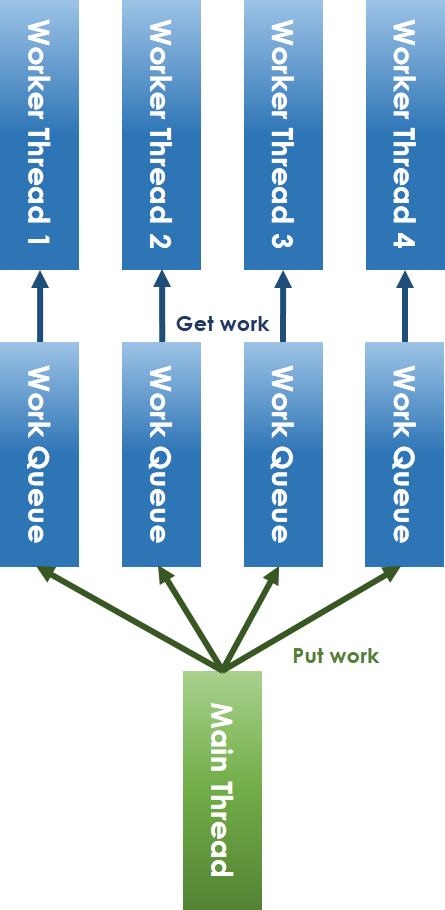#DAY 12
1

## kfifo

kfifo 是 Linux Kernel 中一個 First-In-First-Out 的架構，跟昨天的範例不同，不是一個 Linked List，就只是一段記憶體，但是他是 First-In-First-Out，且允許 `One-Producer-One-Consumer`，是什麼資料結構都無所謂，我們多看例子就是希望可以自己設計或者了解 Concurrent 的程式在不同資料結構中，該如何去作設計

``````struct __kfifo {
unsigned int	in;     //  in: kfifo_put() 塞資料進去的位置
unsigned int	out;    // out: kfifo_get() 取資料出來的位置
unsigned int	mask;   // 有多少個 Element
unsigned int	esize;  // the size of the element managed by the kfifo，雖然是連續的記憶體，但他裡面最小單位是 esize
void		*data;      // 那段連續的記憶體
};

// 總之，在程式中有很多涵式，需要直接操作這段記憶體，所以把他宣告成 union
#define __STRUCT_KFIFO_COMMON(datatype, recsize, ptrtype) \
union { \
struct __kfifo	kfifo; \
datatype	*type; \
const datatype	*const_type; \
char		(*rectype)[recsize]; \
ptrtype		*ptr; \
ptrtype const	*ptr_const; \
}

// Kernel 中不知道為什麼，很喜歡寫出 ptrtype 之類的，然後讓他們再變成宣告的型態，我理解這是一種 Template 的表現 ......
#define __STRUCT_KFIFO(type, size, recsize, ptrtype) \
{ \
__STRUCT_KFIFO_COMMON(type, recsize, ptrtype); \
type		buf[((size < 2) || (size & (size - 1))) ? -1 : size]; \
}

#define STRUCT_KFIFO(type, size) \
struct __STRUCT_KFIFO(type, size, 0, type)
``````

``````// 哪些記憶體已經被使用過了，看到 `recsize` 是指 record size，而 `rectype` 則是指 record type
#define kfifo_recsize(fifo)	(sizeof(*(fifo)->rectype))

// 這邊我不是很懂為什麼要加一？？？？
``````

## 程式碼

### 初始化

``````#define kfifo_init(fifo, buffer, size) \
({ \
typeof((fifo) + 1) __tmp = (fifo); \
struct __kfifo *__kfifo = &__tmp->kfifo; \
__is_kfifo_ptr(__tmp) ? \
__kfifo_init(__kfifo, buffer, size, sizeof(*__tmp->type)) : \
-EINVAL; \
})
``````
``````int __kfifo_init(struct __kfifo *fifo, void *buffer,
unsigned int size, size_t esize)
{
size /= esize;

// 強迫對齊 2 的 N 次方
if (!is_power_of_2(size))
size = rounddown_pow_of_two(size);

fifo->in = 0;
fifo->out = 0;
fifo->esize = esize;
fifo->data = buffer;

if (size < 2) {
return -EINVAL;
}

return 0;
}
``````

### `kfifo_put`

1. `__kfifo_put` -> `kfifo_in`
2. `__kfifo_get` -> `kfifo_out`
3. `kfifo_put` -> `kfifo_in_spinlocked`
4. `kfifo_get` -> `kfifo_out_spinlocked`

3, 4 的修改就是我最開始提到的，以前需要在初始化就把 Lock 傳進來，但現在不用，有需要使用到的時候在傳就好

``````#define	kfifo_put(fifo, val) \
({ \
typeof((fifo) + 1) __tmp = (fifo); \
typeof(*__tmp->const_type) __val = (val); \
unsigned int __ret; \
size_t __recsize = sizeof(*__tmp->rectype); \
struct __kfifo *__kfifo = &__tmp->kfifo; \
if (__recsize) \
__ret = __kfifo_in_r(__kfifo, &__val, sizeof(__val), \
__recsize); \
else { \
__ret = !kfifo_is_full(__tmp); \
if (__ret) { \
(__is_kfifo_ptr(__tmp) ? \
((typeof(__tmp->type))__kfifo->data) : \
(__tmp->buf) \
*(typeof(__tmp->type))&__val; \
smp_wmb(); \
__kfifo->in++; \
} \
} \
__ret; \
})
``````

``````unsigned int __kfifo_in_r(struct __kfifo *fifo, const void *buf,
unsigned int len, size_t recsize)
{
// kfifo_unused 是用來計算還沒使用到的 elements
// 非常單純的 Total Size - Current Size
// (fifo->mask+1) - (fifo->in - fifo->out)
//
// len + recsize 代表的是 [buffer size] + [被使用過的 record]
// 這樣看其實很難理解，因為一般直覺的想法是：
// 「要塞入的資料多長 v.s. 還剩多少空間」
// 但有一個重點，我猜這邊是因為他是環狀的結構，所以還要加上被使用過得資料有多少，只要還沒被使用過，那麼那筆資料最好就不要亂動，所以只能從被使用過得資料開始
if (len + recsize > kfifo_unused(fifo))
return 0;

// 這是一個 helper 涵式，我不知道怎麼形容這個東西 ...，就跟初始化的時候一樣，為了要把 Template 之類的東西丟進來，把什麼東西都變成參數
// 如果限縮 kfifo 的使用條件的話，可以展開成以下的形式
//
// fifo->data[fifo->in & fifo->mask] = (unsigned char)len;
// if(recsize)
//        fifo->data[(fifo->in+1) & fifo->mask] = (unsigned char)len>>8;
__kfifo_poke_n(fifo, len, recsize);

// 較長
// 見以下程式碼
kfifo_copy_in(fifo, buf, len, fifo->in + recsize);
fifo->in += len + recsize;
return len;
}

static void kfifo_copy_in(struct __kfifo *fifo, const void *src,
unsigned int len, unsigned int off)
{
unsigned int size = fifo->mask + 1;
unsigned int esize = fifo->esize;
unsigned int l;

// off 是 fifo->in + recsize
if (esize != 1) {// 一朝回到解放前，終於要算出真正的容量了
off *= esize;
size *= esize;
len *= esize;
}
// min(buffer 大小, ????)
l = min(len, size - off);

// copy 兩次，檢查是否還有要 copy 的東西，因為 l 並不一定是 src 的 size
memcpy(fifo->data + off, src, l);
memcpy(fifo->data, src + l, len - l);
/*
* make sure that the data in the fifo is up to date before
* incrementing the fifo->in index counter
*/
smp_wmb();
}
``````

### `kfifo_in`

``````#define	kfifo_in(fifo, buf, n) \
({ \
typeof((fifo) + 1) __tmp = (fifo); \
typeof(__tmp->ptr_const) __buf = (buf); \
unsigned long __n = (n); \
const size_t __recsize = sizeof(*__tmp->rectype); \
struct __kfifo *__kfifo = &__tmp->kfifo; \
(__recsize) ?\
__kfifo_in_r(__kfifo, __buf, __n, __recsize) : \
__kfifo_in(__kfifo, __buf, __n); \
})

// 沒有 record size 的狀況
unsigned int __kfifo_in(struct __kfifo *fifo,
const void *buf, unsigned int len)
{
unsigned int l;

l = kfifo_unused(fifo);
if (len > l)
len = l;

kfifo_copy_in(fifo, buf, len, fifo->in);
fifo->in += len;
return len;
}
``````

## 所以他的運作原理到底是什麼呢

• 兩個變數: `in` `out`
• 環狀結構## Others

BTW 我自己看了一堆 DP 的題目，結果自己都想不太出來＝＝ 所以看 Concurrent Project 可以學會如何設計嘛，可能要看非常的多才可以@@

0